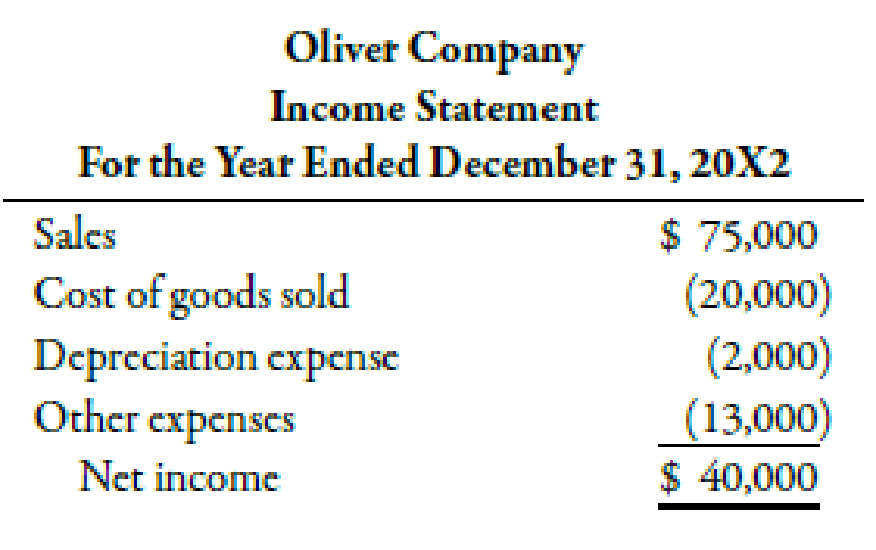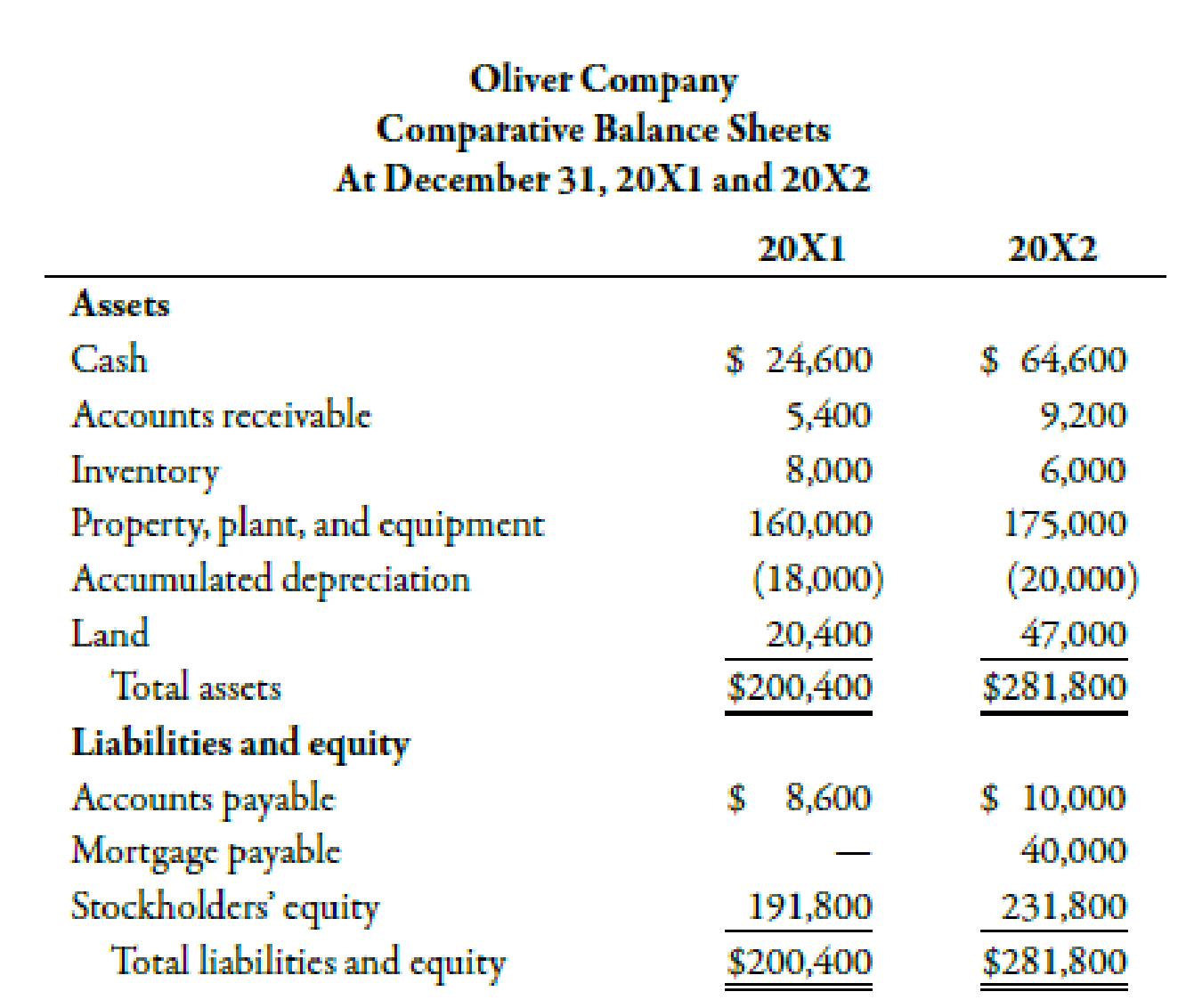Chapter 14, Problem 39E### Managerial Accounting: The Corners...

7th Edition
Maryanne M. Mowen + 2 others
ISBN: 9781337115773

#### Solutions

Chapter
Section### Managerial Accounting: The Corners...

7th Edition
Maryanne M. Mowen + 2 others
ISBN: 9781337115773
Textbook Problem
25 views

# Oliver Company provided the following information for the years 20X1 and 20X2:Required: 1. Calculate the change in cash flows that serves as the control figure for the statement of cash flows. 2. Prepare a schedule that provides operating cash flows for the year 20X2 using the indirect method. 3. Assume that you have all the information provided for Requirement 1 except that you only know the beginning balance of accounts receivable for 20X2. Given this information and assuming that the operating cash flows for 20X2 are $41,000, calculate the ending balance for accounts receivable. 1. To determine Compute the change in cash flows that will serve as the control figure in statement of cash flows. Explanation Change in Cash: The change in cash refers to the amount of difference in the cash inflows and the cash outflows. Use the following formula to calculate the change in cash flows: ChangeinCashFlows=(CashBalanceof20X2CashBalanceof 2. To determine Construct a schedule providing the operating cash flows for the year 20X2 using the indirect method. 3. To determine Compute the ending balance for accounts receivable assuming the operating cash flows for 20X2 as$41,000.

### Still sussing out bartleby?

Check out a sample textbook solution.

See a sample solution

#### The Solution to Your Study Problems

Bartleby provides explanations to thousands of textbook problems written by our experts, many with advanced degrees!

Get Started

#### Find more solutions based on key concepts